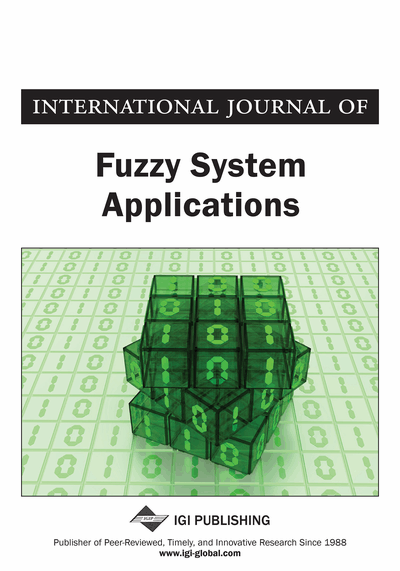# MAGDM Problems with Correlation Coefficient of Triangular Fuzzy IFS

John P. Robinson (Bishop Heber College, Tamilnadu, India) and Henry Amirtharaj E.C. (Bishop Heber College, Tamilnadu, India)
DOI: 10.4018/IJFSA.2015010101

## Abstract

Correlation coefficient of Intuitionistic Fuzzy Set (IFS), Interval valued IFS, Triangular IFS and Trapezoidal IFS are already present in the literature. This paper proposes the correlation coefficient for Triangular Fuzzy Intuitionistic Fuzzy set (TrFIFS). The method on uncertain Multiple Attribute Group Decision Making (MAGDM) problems based on aggregating intuitionistic fuzzy information is investigated for TrFIFSs. The Triangular Fuzzy Intuitionistic Fuzzy Ordered Weighted Averaging (TrFIFOWA) operator is proposed for TrFIFSs and the Triangular Fuzzy Intuitionistic Fuzzy Ordered Weighted Geometric (TrFIFOWG) operator is utilized for decision making models where expert weights are completely unknown. Based on these operators and the correlation coefficient defined for the TrFIFSs, new decision making models are proposed with numerical illustrations. Some comparisons are also made with existing ranking methods for validity.
Article Preview

## Introduction

Decision making is the study of identifying and choosing alternatives based on the values and preferences of the decision maker. Making a decision implies that there are alternative choices to be considered, and in such cases many of these alternatives as possible should be identified and the one that best fits with our goals, objectives, desires, values, should be chosen (Kahraman, 2008). Decision making should start with the identification of the decision maker(s) and stakeholder(s) in the decision, reducing possible disagreement about problem definition, requirements, goals and criteria. It is very important to make a distinction between the cases where we have a single or multiple criteria. When a decision problem has a single criterion or a single aggregate measure, then the decision can be made implicitly by determining the alternative with the best value of the single criterion or aggregate measure. When a decision problem has a finite number of criteria or multiple criteria, and the number of the feasible alternatives (the ones meeting requirements) is infinite, and then the decision problem belongs to the field of multiple criteria optimization. Also, techniques of multiple criteria optimization can be used when there are a finite number of feasible alternatives, but are given only in implicit form. This research work focuses on decision making problems when the number of the criteria (attribute) and alternatives is finite, and the alternatives are explicitly given. Problems of this type are called Multi Attribute Decision Making (MADM) problems.

Consider a multi-attribute decision making problem with m criteria and n alternatives. Let C1,...,Cm and A1,...,An denote the criteria and alternatives, respectively. A standard feature of multi-attribute decision making methodology is the decision table as shown in the following. In this table each row belongs to a criterion and each column describes the performance of an alternative. The score aij describes the performance of the alternative Aj against the criterion Ci. For the sake of simplicity it is assumed that a higher score value means a better performance, as any goal of minimization can easily be transformed into a goal of maximization.

Here the weights wi reflects the relative importance of criteria Ci to the decision, and is assumed to be positive. These weights are usually determined on subjective basis and they represent the opinion of a single decision maker or synthesize the opinions of a group of experts using a group decision technique. The values x1, ..., xn are associated with the alternatives in the decision table, and are the final ranking values of the alternatives Aj. Usually, a higher ranking value means a better performance of the alternative; hence the alternative with the highest ranking value is the best of the alternatives. Group decision is usually understood as aggregating different individual preferences on a given set of alternatives to a single collective preference. It is assumed that the individuals participating in making a group decision face the same common problem and are all interested in finding a solution. A group decision situation involves multiple actors (decision makers), each with different skills, experience and knowledge related to different aspects (criteria) of the problem. In a correct method for synthesizing group decisions, the competence of different actors to different professional fields has also to be considered. It is assumed that each actor considers the same sets of alternatives and criteria. It is also assumed that there is a special actor with authority to establish consensus rules and determine voting powers to group members on different criteria. Many researchers call this entity the Supra Decision Maker (SDM). The final decision is derived by aggregating (synthesizing) the opinions of group members according to rules and priorities defined by the SDM. There are several approaches to extend the basic multi attribute decision making techniques for group decision. Consider a decision problem with l group members (decision makers) D1,..,Dl, n alternatives A1,...,An and m criteria C1,..,Cm. In case of a factual criterion, the evaluation scores must be identical for any alternative and any decision maker, while subjective (judgmental) criteria can be evaluated differently by each decision maker. In this work, Multiple Attribute Group Decision Making (MAGDM) problems with applications of different classes of aggregation operators, and correlation coefficient in triangular fuzzy IFS environment as the ranking method have been concentrated upon.

## Complete Article List

Search this Journal:
Reset
Open Access Articles: Forthcoming
Volume 9: 4 Issues (2020): Forthcoming, Available for Pre-Order
Volume 8: 4 Issues (2019): 3 Released, 1 Forthcoming
Volume 7: 4 Issues (2018)
Volume 6: 4 Issues (2017)
Volume 5: 4 Issues (2016)
Volume 4: 4 Issues (2015)
Volume 3: 4 Issues (2013)
Volume 2: 4 Issues (2012)
Volume 1: 4 Issues (2011)
View Complete Journal Contents Listing